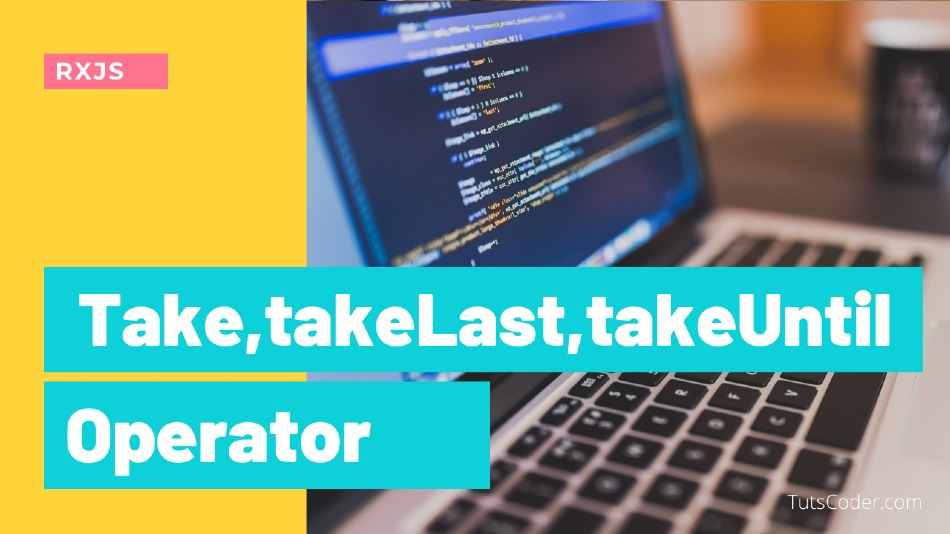In this tutorial, we will learn about take, takeLast, and takeUntill operator of RXJS.

## Take Operator

The take operator is a Filtering Operator. Filtering Operators allows you to select how and when to accept values emitted from Observables.

It allows you to specify a maximum number of values you want to receive from an Observable

Take() Emits only the first count values emitted by the source Observable.

Using take we can take only data which we required from observable

``````randomNames = ["jigar", "Rahul", "Seema", "Aadi", "Megh"];

const nameSource = from(this.randomNames);

nameSource.pipe(take(4)).subscribe((res) => {
console.log(res);
});``````

Output:

``````jigar
Rahul
Seema

Use Cases:

It can be used in situations where we want to limit how many user-produced events (fromEvent) we want to handle, for example, the first time the user clicks in our app

## takeLast Operator

takeLast() Emits only the last count values emitted by the source Observable.

``````randomNames = ["jigar", "Rahul", "Seema", "Aadi", "Megh"];

const nameSource = from(this.randomNames);

nameSource.pipe(takeLast(4)).subscribe((res) => {
console.log(res);
});
``````

Output:

``````Rahul
Seema
Megh``````

## TakeUntil Operator

takeUntil Emits the values emitted by the source Observable until a notifier Observable emits a value.

Normally we can say takeUntill operator is based on condition and Takes condition as observable

For Example, we want a timer that will stop after some specific seconds, lets see how we can do this using takeUntill Operator:

``````const source = interval(1000);
//Will Stop after 6 sec
let condition = timer(6000);

source
.pipe(
map((res) => "Number " + res),
takeUntil(condition)
)
.subscribe((res) => {
console.log(res);
});``````

Output:

``````1
2
3
5
6``````